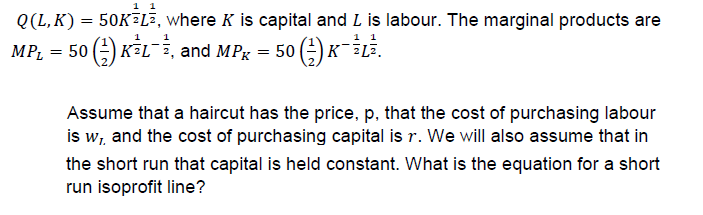# 0(L, K) = 50K2Lī, where K is capital and L is labour. The marginal products areMPL 5KLi, and MP 50-iLi.Assume that a haircut has the price, p, that the cost of purchasing labouris wi and the cost of purchasing capital is r. We will also assume that inthe short run that capital is held constant. What is the equation for a shortrun isoprofit line?

Question
15 viewshelp_outlineImage Transcriptionclose0(L, K) = 50K2Lī, where K is capital and L is labour. The marginal products are MPL 5KLi, and MP 50-iLi. Assume that a haircut has the price, p, that the cost of purchasing labour is wi and the cost of purchasing capital is r. We will also assume that in the short run that capital is held constant. What is the equation for a short run isoprofit line? fullscreen
check_circle

Step 1

Production technologies or production function defines a relationship between the output and inputs. We are given the production function as follows.

Step 2

We are given the cost of purchasing capital=r

And, the cost of purchasing labor=w.

Thus, to hire L units of labor and K units of capital, the cost would be,

Step 3

Profit is defined as total revenue net of total costs. Now, we write the profit function (π) as per the given infor...

### Want to see the full answer?

See Solution

#### Want to see this answer and more?

Solutions are written by subject experts who are available 24/7. Questions are typically answered within 1 hour.*

See Solution
*Response times may vary by subject and question.
Tagged in